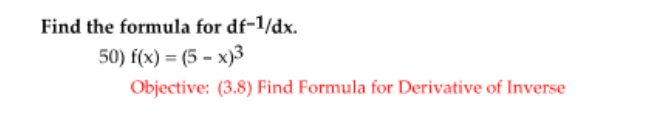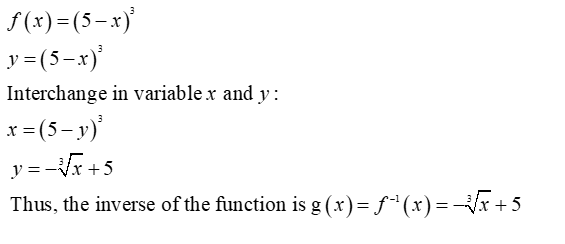# Find the formula for df-1/dx.50) f(x) = (5 - x)3Objective: (3.8) Find Formula for Derivative of Inverse

Question
2 views

Can i get help step by step with this problem?help_outlineImage TranscriptioncloseFind the formula for df-1/dx. 50) f(x) = (5 - x)3 Objective: (3.8) Find Formula for Derivative of Inverse fullscreen
check_circle

Step 1

First,, find the inverse of the function f(x)=(5–x)3...

### Want to see the full answer?

See Solution

#### Want to see this answer and more?

Solutions are written by subject experts who are available 24/7. Questions are typically answered within 1 hour.*

See Solution
*Response times may vary by subject and question.
Tagged in